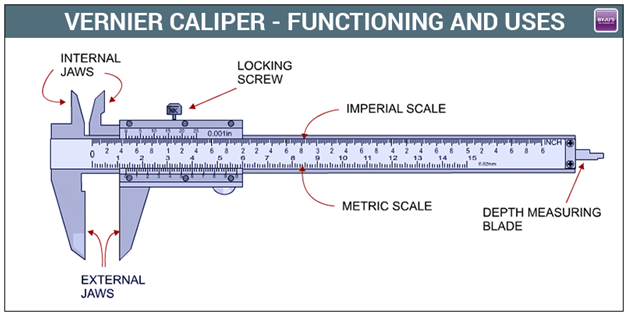# What is the Least Count of Vernier Caliper

The formula of Vernier calliper least counts is determined by dividing the smallest reading of the main scale with the total number of vernier scale divisions. The LC of vernier calliper is the difference between one smallest main scale reading and one smallest vernier scale reading of 0.1 mm 0r 0.01 cm.

The lowest count error is the error correlated with the instrument’s resolution. It can have graduations at 1 mm division scale spacing or interval if we use a metre scale shape calculation of length. A Vernier calliper scale can have a minimum count of 0.01 mm, while a micrometre can have a minimum count of 0.001 mm.The Least Count measuring instrument is determined mathematically by calculating the main scale reading by the cumulative number of divisions on the main scale. And if the method has a Secondary Size, too. Then the ratio of the major scale L.C. is instrument LC. And the number of sub-scale divisions.(43)(7)